Latest Banking jobs   »

# Quantitative Aptitude Quiz For IBPS RRB PO/Clerk Mains 2022- 2nd September

Directions (1-5): The following questions are accompanied by two statements (A) and (B). You have to determine which statement(s) is/are sufficient /necessary to answer the questions
(a) if the Statement ‘A’ alone is sufficient to answer the question but the Statement ‘B’ alone is not sufficient
(b) if the Statement ‘B’ alone is sufficient to answer the question but the Statement ‘A’ alone is not sufficient
(c) if both Statement ‘A’ and ‘B’ together are needed to answer the question
(d) if either the Statement ‘A’ alone or Statement ‘B’ alone is sufficient to answer the question
(e) if you cannot get the answer from both the Statements together

Q1. Find the value of 8y² (y + 3x) + 8x² (x + 3y)
A) Product of x and y is equal to 6
B) Sum of inverse of x and y is equals to 5/6

Q2. If X, Y and Z together can complete the work in 8 days, find in how many days Y alone can complete the work?
A) X and Y together can complete the work in 12 days while Z is 25% less efficient than ‘X’.
B) Z is 50% more efficient than ‘Y’ who is 100% less efficient than ‘X’.

Q3. What will be the speed of boat if A is the starting point and B is the destination point?
A) Man go to a point B and come back to initial point A in total 12 hours while the ratio of speed of boat to speed of stream is 3 : 2.
B) Man reached at mid-point of A and B in 5 hour and then go to point B and come back to mid-point of A and B in total 6 hours while speed of stream is 10 km/hr.

Q4. Three numbers are co-prime to each other. Find the sum of these numbers?
A) Product of first two number is 286 while the product of last two numbers is 470.
B) Sum of first two number is 35 while the sum of last two number is 57.

Q5. Find the sum of digits of given two-digit number?
A) If we interchange the numbers then the new number formed is greater than the original number by 9.
B) If we interchange the number then product of new number and original number is 1462.

Directions (Q6-10): Each of the questions below consists of a question and two statements numbered I and II given below it. You have to decide whether the data provided in the statements are sufficient to answer the question. Read both the statements and give answer
(a) if the data in statement I alone are sufficient to answer the question, while the data in statement II alone are not sufficient to answer the question.
(b) if the data in statement II alone are sufficient to answer the question, while the data in statement I alone are not sufficient to answer the question.
(c) if the data either in statement I alone or in statement II alone are sufficient to answer the question.
(d) if the data in both statement I and II together are not sufficient to answer the question.
(e) if the data in both statement I and II together are necessary to answer the question.

Q6. What is the speed of a boat in still water ?
I. The boat covers a distance of 160 km in 8 hours while running upstream.
II. It covers the same distance in 4 hours while running downstream.

Q7. How many boys are there in the class ?
I. The number of girls is 25% of the number of boys.
II. The ratio of the number of boys to that of girls is 5 : 7.

Q8. What is the value of a two-digit number ?
I. The sum of the digits of the number is 13.
II. The digit at the tens place is 160% of the digit at the units place.

Q9. What is the area of a square ?
I. The diagonal of the square is 8√2 m.
II. The perimeter of the square is 32 m.

Q10. What is the speed of a train ?
I. The train crosses a pole in 16 seconds.
II. The train crosses a platform of equal length in 54 seconds.

Directions (11–15): In the given questions, two quantities are given, one as ‘Quantity I’ and another as ‘Quantity II’. You have to determine relationship between two quantities and choose the appropriate option

Q11. Quantity I – Solve the question according to given data in table.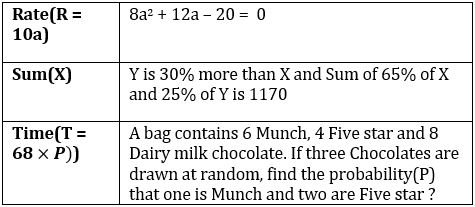Find the compound interest of T/2 years compounding annually.
Quantity II – 184 Rs.
(a) Quantity I > Quantity II
(b) Quantity I < Quantity II
(c) Quantity I ≥ Quantity II
(d) Quantity I ≤ Quantity II
(e) Quantity I = Quantity II or no relation

Q12. Quantity I – Length of main diagonal of a cube is 60√3 cm, if cube is melted in the form of smaller cube with side of 4 cm each or cube is melted in the form of cuboids of each having length of 8 cm, width 5 cm & height 2 cm. Find the difference between total surface area of all smaller cubes & all cuboids formed (in m²)?
Quantity II – 3.48 m²
(a) Quantity I > Quantity II
(b) Quantity I < Quantity II
(c) Quantity I ≥ Quantity II
(d) Quantity I ≤ Quantity II
(e) Quantity I = Quantity II or no relation

Q13.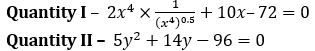(a) Quantity I > Quantity II
(b) Quantity I < Quantity II
(c) Quantity I ≥ Quantity II
(d) Quantity I ≤ Quantity II
(e) Quantity I = Quantity II or no relation

Q14.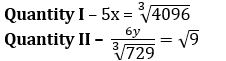(a) Quantity I > Quantity II
(b) Quantity I ≤ Quantity II
(c) Quantity I ≥ Quantity II
(d) Quantity I < Quantity II
(e) Quantity I = Quantity II or no relation

Q15.

Quantity I – 18x²-15x+3=0
Quantity II –24y²+20y–4=0
(a) Quantity I > Quantity II
(b) Quantity I ≤ Quantity II
(c) Quantity I ≥ Quantity II
(d) Quantity I < Quantity II
(e) Quantity I = Quantity II or no relation

Solutions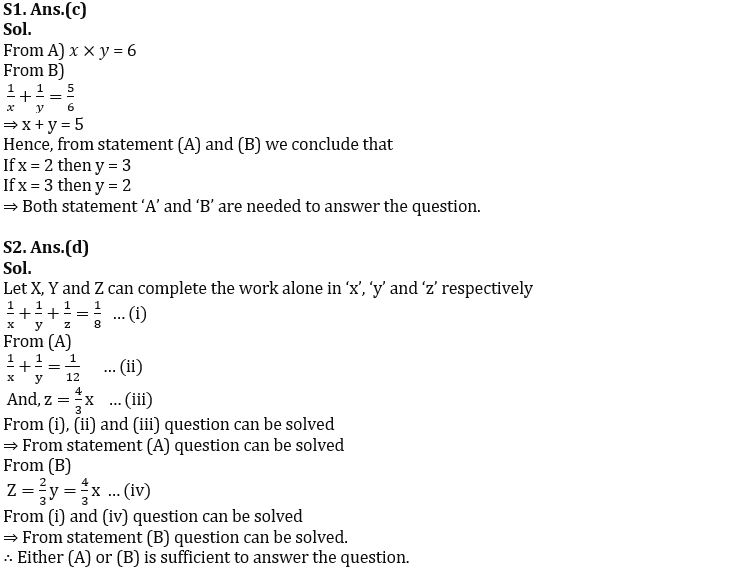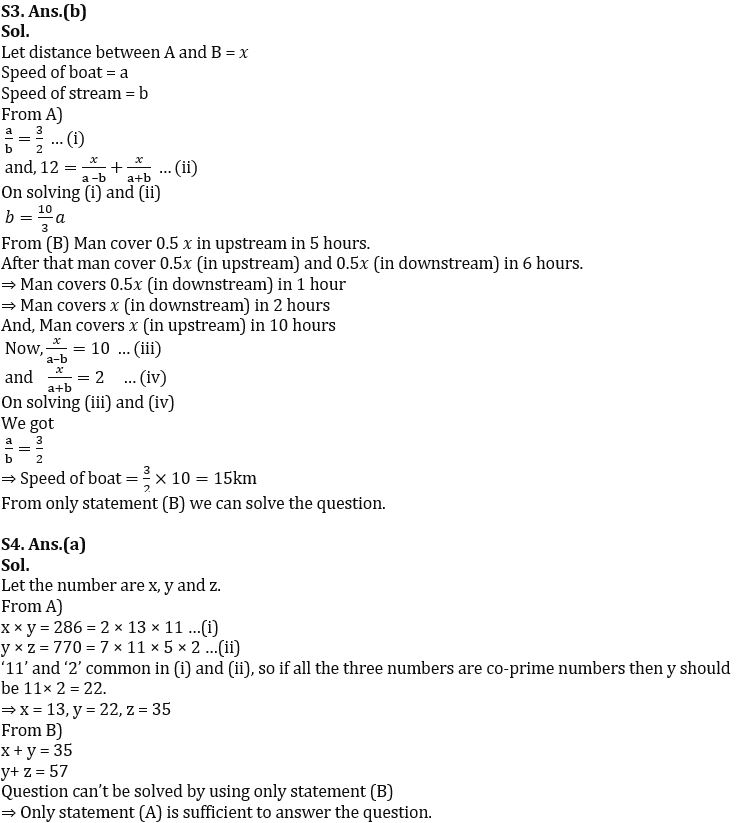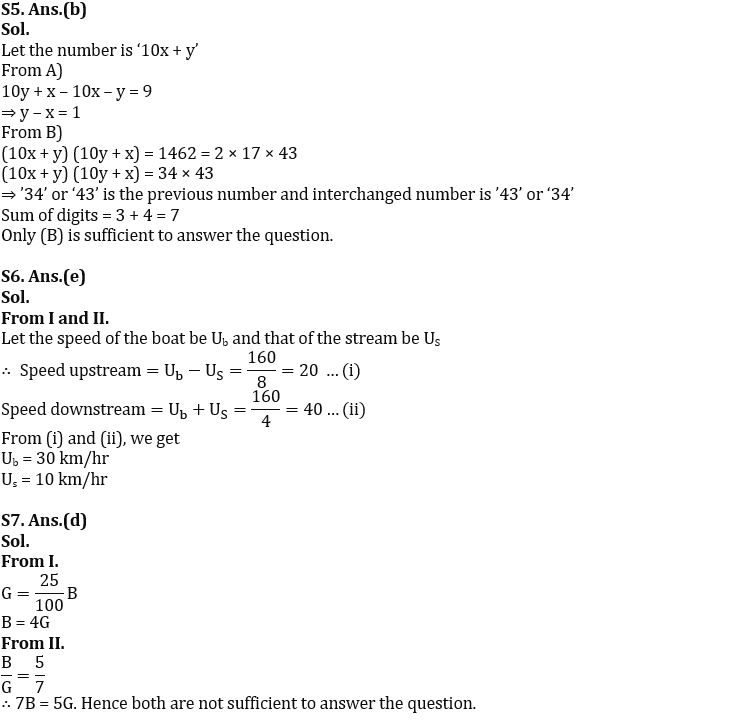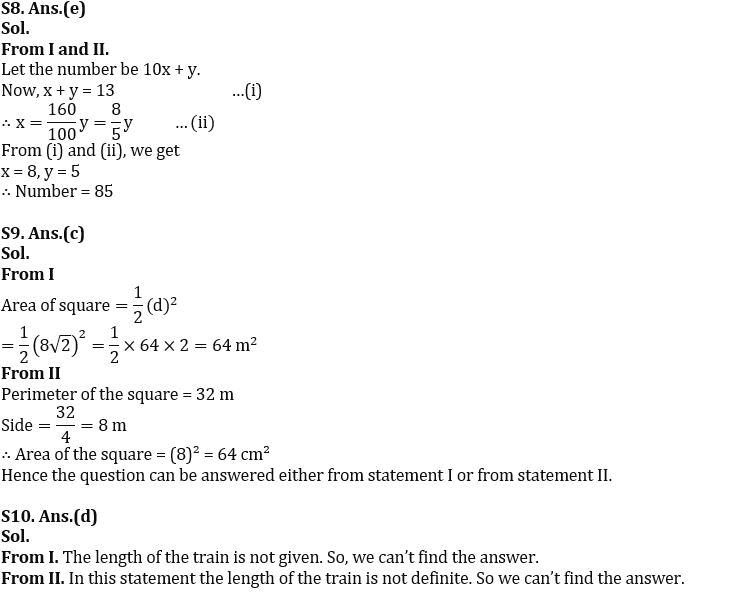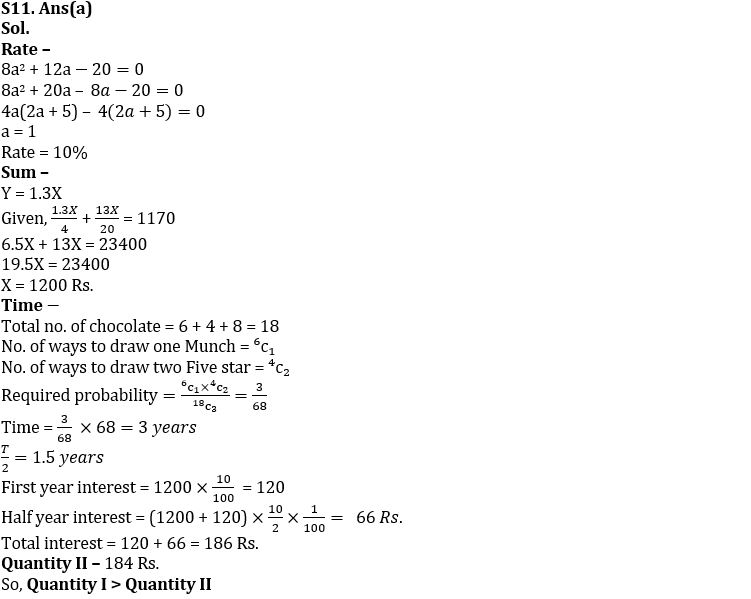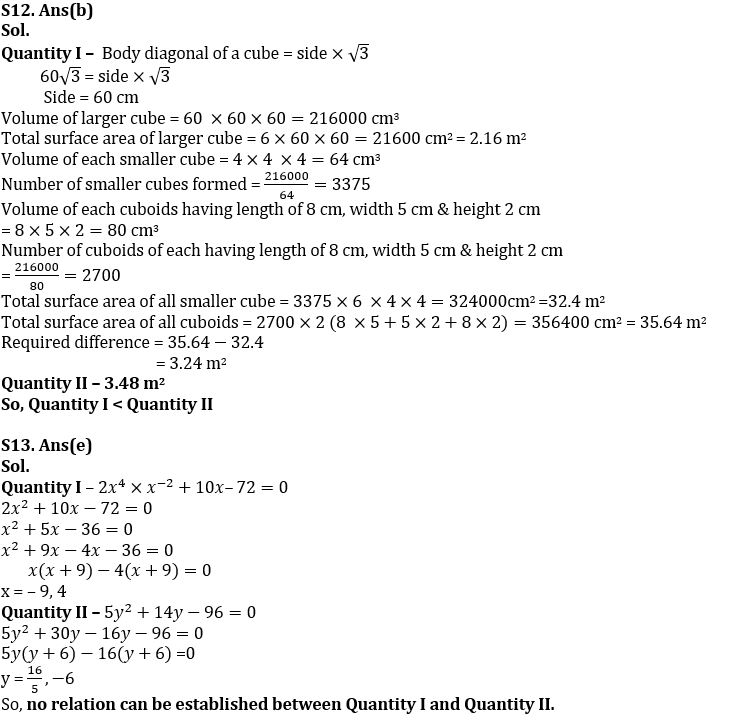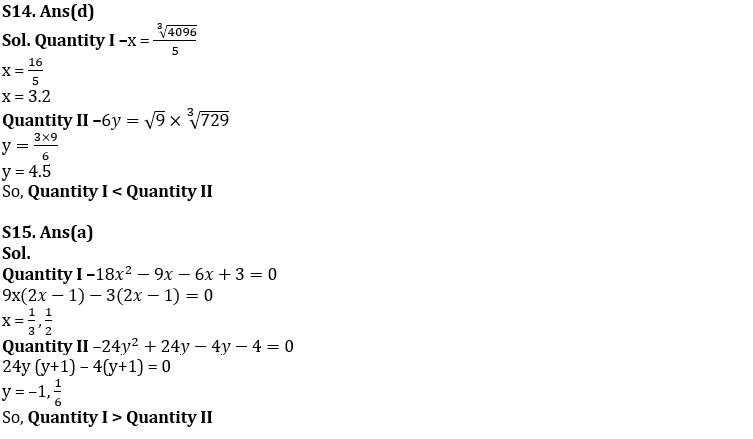#### Congratulations!Download Hindu Review of October 2021: Free PDF